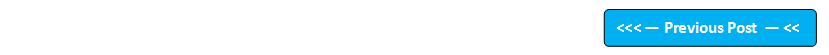Google+

## Blog Pages

### 173. Using 'length' array attribute with 3 dimensional arraysPre-requisites -

1. Post # 48 Arrays
2. Post # 92 'length' array attribute

Assuming that you have understood the array concepts from the above pre-requisite posts, I would like to explain how to use 'length' attribute to calculate the number of 2 dimensional arrays, rows and number of columns in a 3 dimensional array.

Example of a 2 dimensional array -

int a[][][] = new int;

Visual representation of the array -

To calculate the number of 2 dimensional arrays in this 3 dimensional array use -> a.length

To calculate the number of rows in the first 2 dimensional array of the 3 dimensional array use -> a.length

To calculate the number of columns in the first row of the first 2 dimensional array of the 3 dimensional array use -> a.length

To calculate the number of columns in the second row of the first 2 dimensional array of the 3 dimensional array use -> a.length

To calculate the number of columns in the third row of the first 2 dimensional array of the 3 dimensional array use -> a.length

To calculate the number of rows in the second 2 dimensional array of the 3 dimensional array use -> a.length

To calculate the number of columns in the first row of the second 2 dimensional array of the 3 dimensional array use -> a.length

To calculate the number of columns in the second row of the second 2 dimensional array of the 3 dimensional array use -> a.length

To calculate the number of columns in the third row of the second 2 dimensional array of the 3 dimensional array use -> a.length

Lets implement this on Eclipse IDE -

1. Launch Eclipse IDE, open Java Project 'Project 018' and create a new Java Class 'Class2' with main( ) method as shown below -

2. Write the following code to print arrays, rows and columns as shown below -

3. Run the java class 'Class2' and observe that the calculation got printed in the console as shown below -

This is how, we use the length attribute in a two dimensional array.

How to work with 'char' data type arrays will be explained in the next post.

Please comment below to feedback or ask questions.